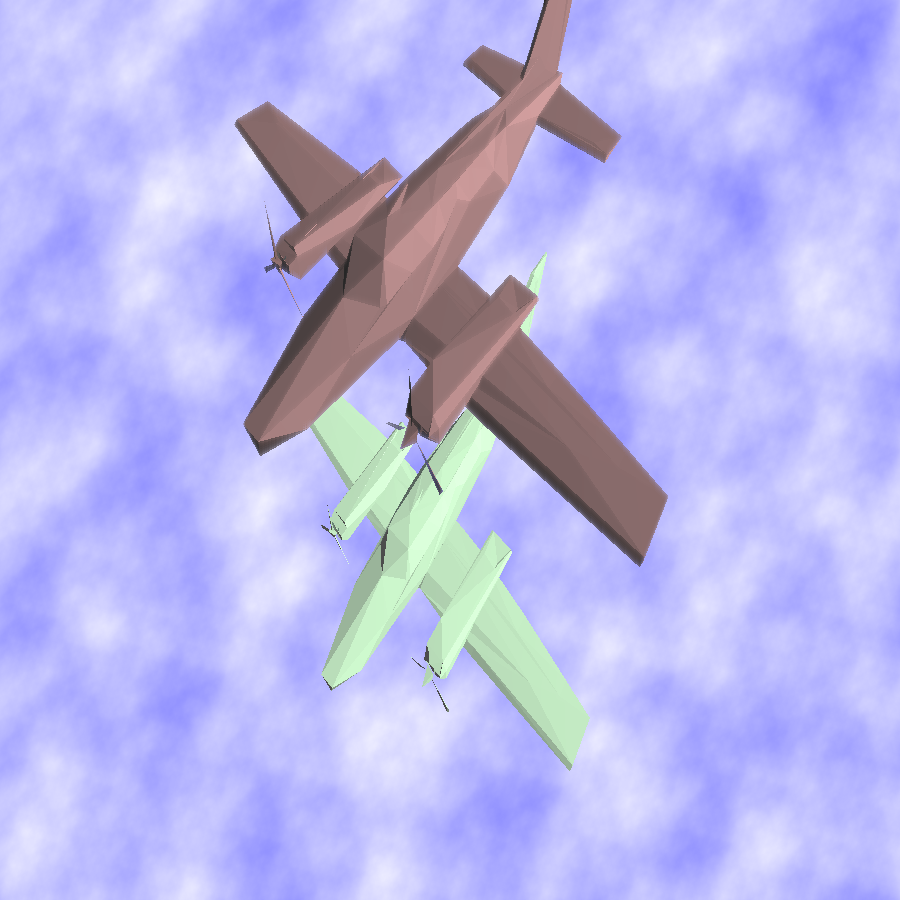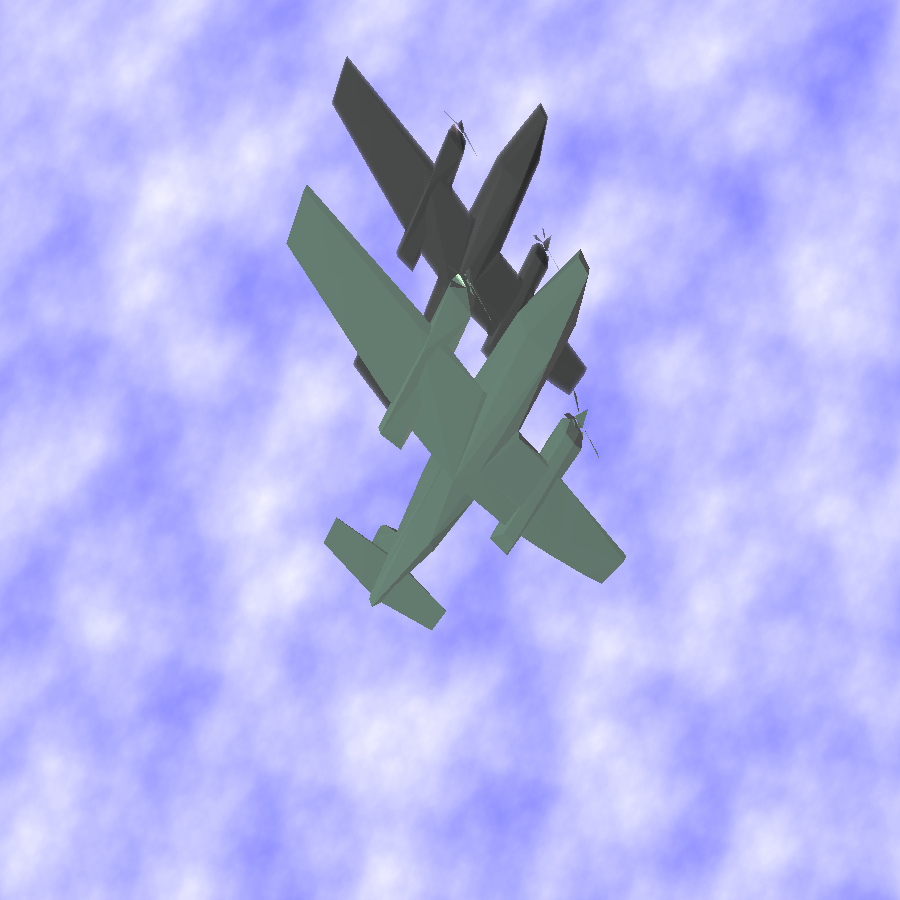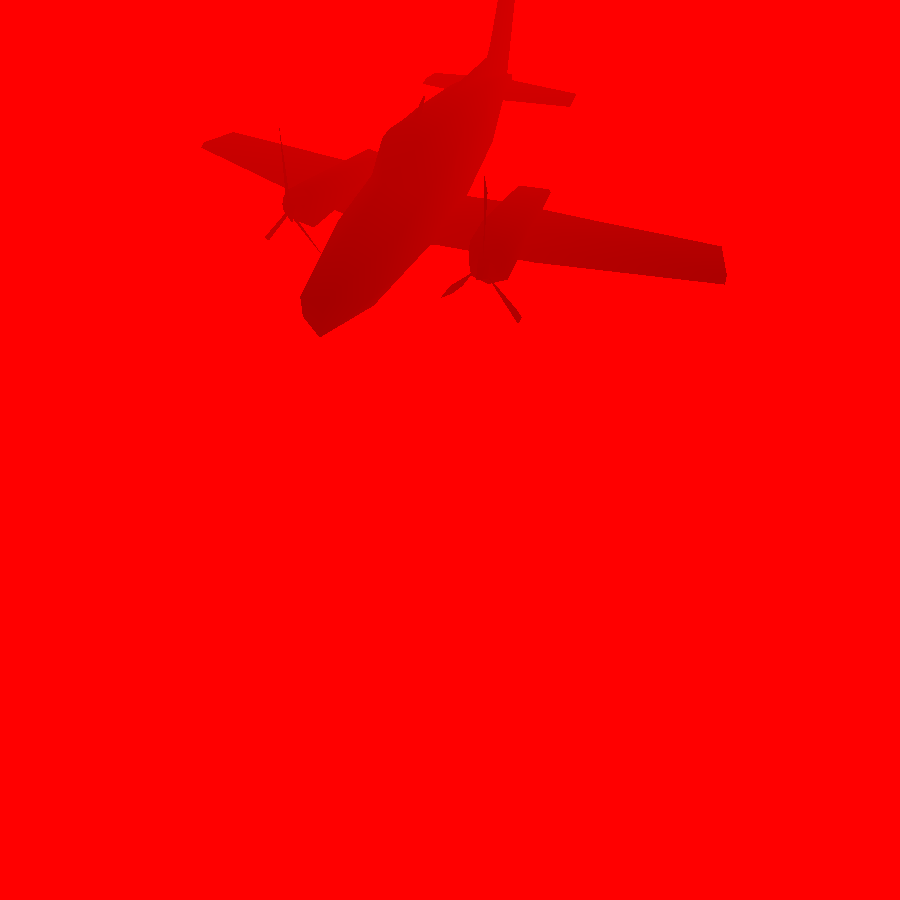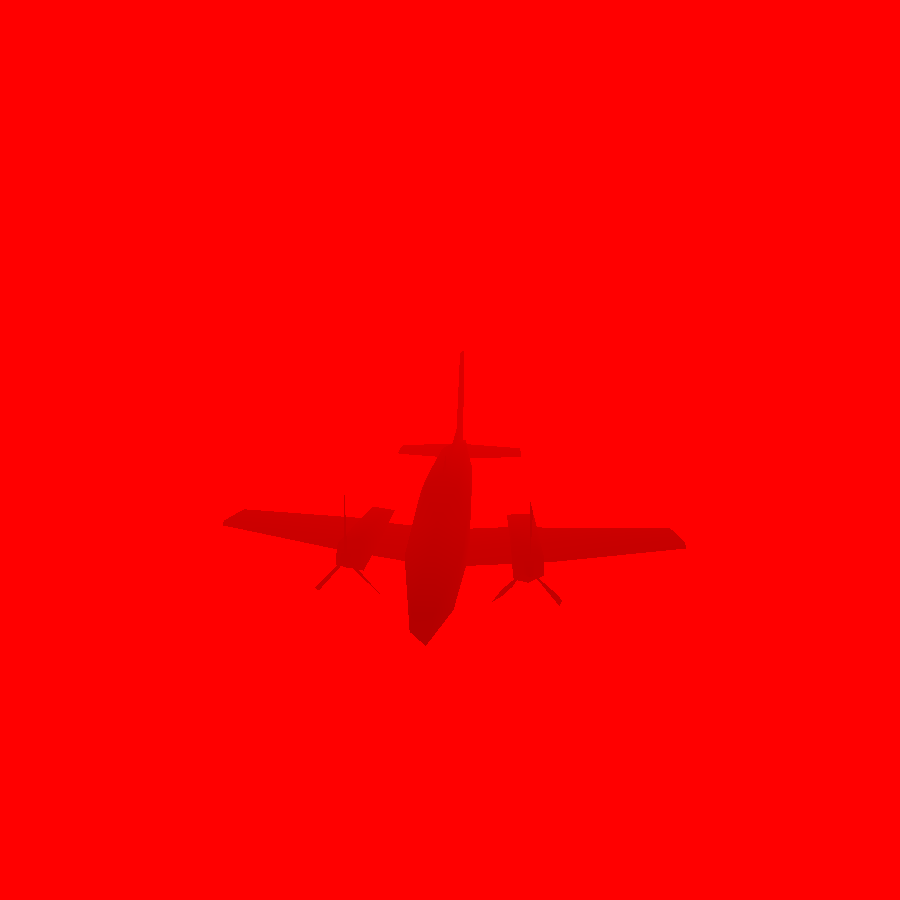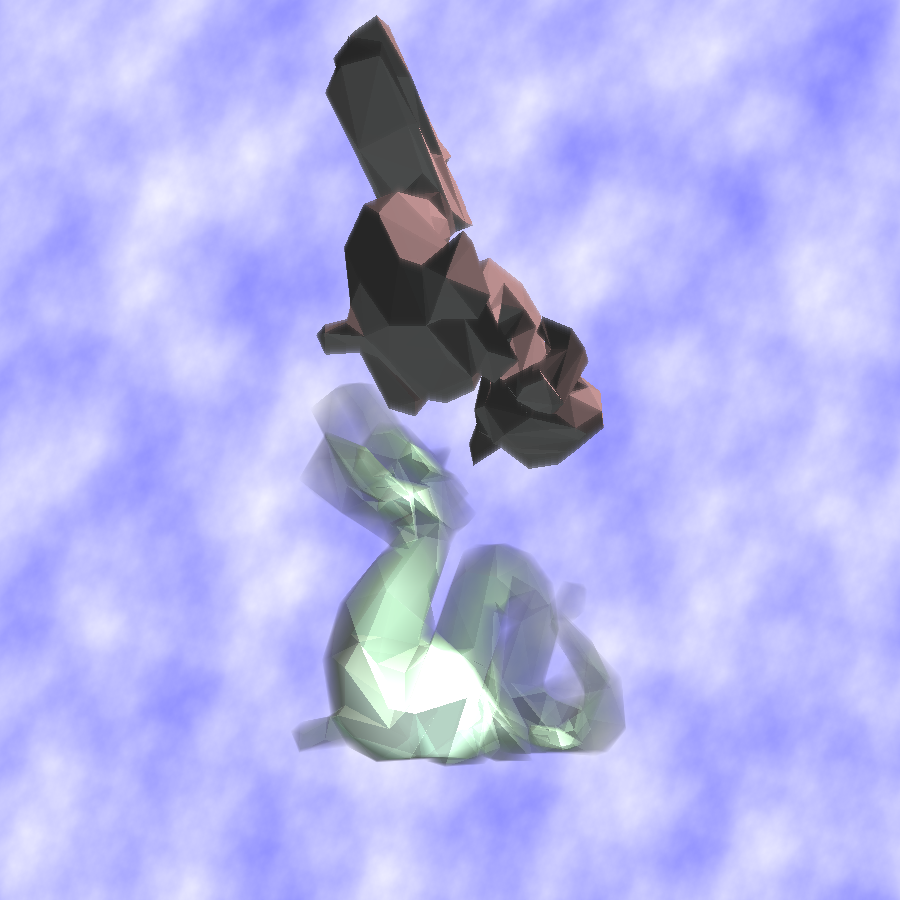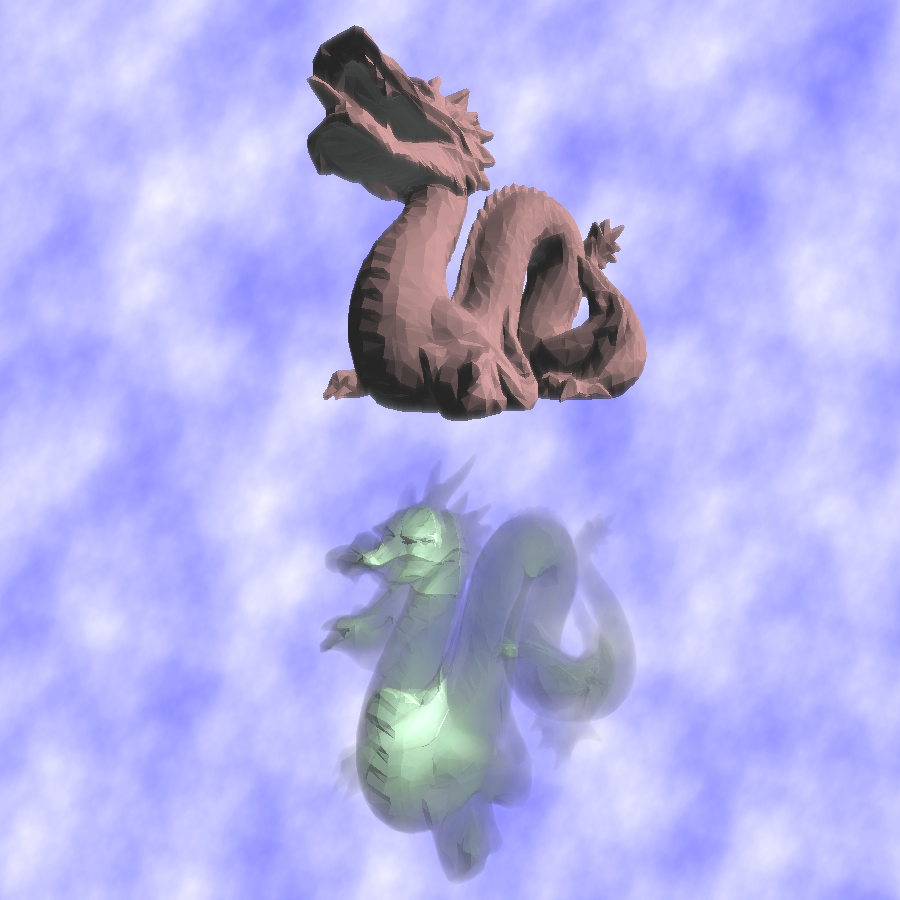# Fractal Rendering!

## Introduction to the Mandelbrot Set

The Mandelbrot Set is a set of points in the complex plane which take on an iconic and recognizeable shape when drawn as an image. The set is represented by a recurrence relation:

Zn+1 = Zn2 + c

Where Z0 = 0 + 0i and c is point in the complex plane. If Zn does not approach infinity for arbitrarily large values of n, then c is considered an element of the Mandelbrot Set. Otherwise, if Zn approaches infinity, then c is not a part of the Mandelbrot Set.

As you may imagine, it is non-trivial to quickly determine whether an infinity series is divergent or not. However, there are some useful facts that make approximating the series quite easy. For example, any complex point whose distance from the origin is greater than or equal to 2 is definitely not an element of the set. We also know (thanks to the nature of the recurrence relation) that, if while calculating values of Zn for some point c, we encounter a point which is not in the set, then c is not in the set either.

## Computing the Image

One of the most common and simple to implement algorithms used for drawing the Mandelbrot Set is based on these facts. It is called the escape-time algorithm, and these are the basic steps required to implement it:

1. Establish a maximum iteration count. This number is sometimes referred to as the Dwell Limit. The detail of our rendered image will depend on how high the limit is, but higher limits will produce longer render times.
2. Iterate over every point c in the complex plane that we would like to render. The rectangular region from -2.5 to 1 on the real axis and from -1 to 1 on the imaginary access encompases all of the points in the Mandelbrot Set, and is the most iconic viewport to render.
3. Start with Z0 = 0 + 0i. Next, Z1 = c and Z2 = c2 + c. Continue calculating values of Z in this way until we either find a point Zn which is farther than two units away from the origin, or until we have reached our maximum iteration count.
• If we reached the dwell limit, then for our approximate rendering we are assuming that the point c is inside the Mandelbrot Set. Choice a color to represent the set, and draw the point c with this color.
• If we found a value Zn for which |Zn| >= 2 (the point is two or more units away from the origin), then we have determined that c is not within the set. Now, use the number of iterations it took to determine this to pick a color for the point c. Two common ways of doing this are using a gradient or by using the iteration count as a lookup index in a texture.

The crux of the escape-time algorithm is choosing the Dwell Limit. The value that is chosen will not only affect the shape of the image drawn, but also how it is colored.

While the algorithm is easy to implement, it has a number of flaws. One of the most promiment is color banding - since we deal with a discrete number of possible iterations that can be run before the Dwell Limit is reached, there are similarily a discrete number of colors which will be drawn by the algorithm. In some circumstances, the borders between colors in the image can become very promiment. There are other algorithms which address this issue, but they are outside of the scope of this website.

## Other Fractals

There are a number of different fractals which are based on the Mandelbrot Set, often with some simple alteration to the Recurrence Relation which produces different output images.

• ### The Mandelbar Set

This fractal is also sometimes called the Tricorn. It is formed by raising Z to the negative 2nd power in the Recurrence Relation, like so: Zn+1 = Zn-2 + c
• ### The Multibrot Sets

There are a number of different fractals which are collectively referred to as the Multibrot Sets. Each of these fractals has the recurrence relation Zn+1 = Znf + c where f is some arbitrary constant. For the special case f = 2, we get the traditional Mandelbrot Set. For the case f = -2, we get the Tricorn.
• ### Burning Ship Fractal

This fractal is formed by setting each component of Zn to its absolute value before squaring, that is: Zn+1 = abs(Zn)2 + c. It takes on the appearance of a burning and sinking ship, hence the name. It's details are much more chaotic than some of the other Mandelbrot-related Sets.
• ### Buddhabrot

This fractal is the most complex and the most difficult to render of those listed here. The Buddhabrot fractal is formed by taking a large overview render of the Mandelbrot Set and drawing the paths of each point which is found to be outside the set as it iterates towards infinity.

## My Implementation

During the course of this project, I developed a number of different implementations for draw the Mandelbrot Set and other related fractals. I first developed a simple software version in C++ which could output to bitmap images. I then converted the software version to CUDA - the CUDA implementation was effective because of house easily the calculation of the Recurrence Relation lends itself to the multithreaded model, and the implementation was significantly faster than the software version.

However, the limitations of the CUDA model itself - namely, it's dependance on recent and nVidia branded hardware, and the finickiness of its compiler - caused me to disfavor this implementation. I instead opted for an OpenGL implementation, using a fragment shader to calculate individual values of the fractal and displaying them on a full-screen quad. This implementation proved very effective, and on a modern and powerful GPU I was able to traverse and explore the different fractals in real-time.

My implementation consisted of a fragment shader for the traditional Mandelbro Set, Mandelbar Set, Multibrot Sets for f = 3 and f = 4, and the Burning Ship Fractal. For each fractal, I also developed 4 different levels of multisampling. The higher levels of multisampling greatly cripple the performance of the rasterizer but provide smooth images with little or no aliasing artifacts.

In addition to the shader-based version, I repurposed the software version for doing long-term headless renders, ie. for video output or exceptionally large and/or highly detailed rasterizations. I also used this software version for doing renderings of the Buddhabrot set, which is too complex to render with a GLSL shader.

# Software Deep Depth Buffer Transparency

## Concept

Hardware graphics APIs like OpenGL and DirectX make use of Z-Buffers to handle occlusion tests. These depth tests work by making each write to a discrete pixel location an atomic operation, and during each write a binary depth-check is performed. This makes it so that at any given pixel on the screen, the color shown is from the nearest scene geometry item - a system which is ideal in most cases except for when dealing with transparency. The z-buffer system allows for geometry to be rendered in an arbitrary order, which is appropriate for the multi-threaded model best suited for graphics processing. However, it is impossible to perform a true calculation of transparency blending without knowledge of what order objects are sorted in, and such information is not available in a z-buffer system.

However, by using a system which saves information about each non-opaque fragment which is drawn at a given pixel location, it is possible to peform an accurate transparency blending calculation. A system which stores information about more than just one fragment Such a system would be difficult to implement on graphics hardware using an API such as OpenGL since the z-buffer system is so deeply ingrained in those interfaces.

Deep depth buffering is much easier to implement using serial architecture (ie. for a software rasterizer) but such a system is not well suited for rendering large amounts of scene geometry.

Therefore, the ideal system would be one that exists primarly on the GPU and which uses the CPU for computing depth-independant transparency only when the GPU is unable to.

## My Implementation

For the second part of my project, I implemented a combined software-hardware scene rendering system which renders a 3d scene using OpenGL with some select components rendered simultaneously on the CPU and then merged with the GPU scene.

Each half of the scene is rendered using the same view and projection transforms. The two individual depth buffers are then used to merge the two renders so they appear to have been rendered simultaneously.

The most blatant flaw in this system is the crippling slowness of the CPU part of the rasterizer. Possible future work includes optimizing the software renderer and/or providing a CUDA-based rasterizer if deep depth buffering can be adequately implemented.

# Screenshots

Click an image to view larger. With the exception of the Buddhabrot renders, all images shown are direct output from the rasterizer. The Buddhabrot renders were colorized from grayscale images.

## Mandelbrot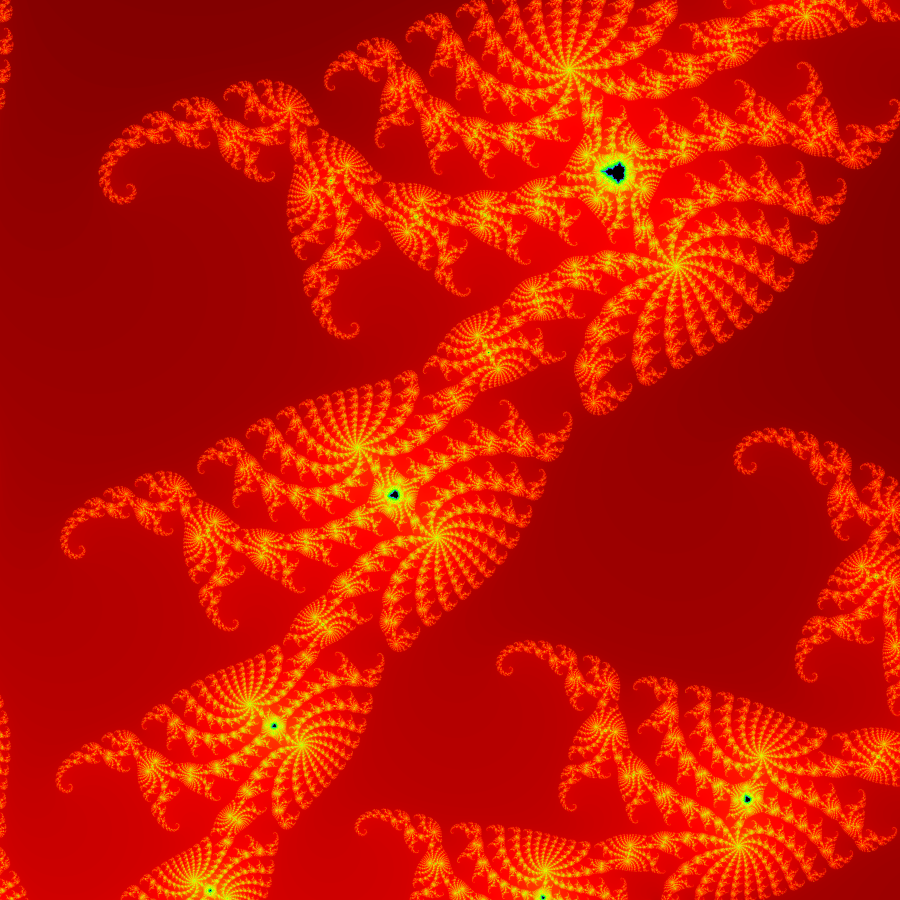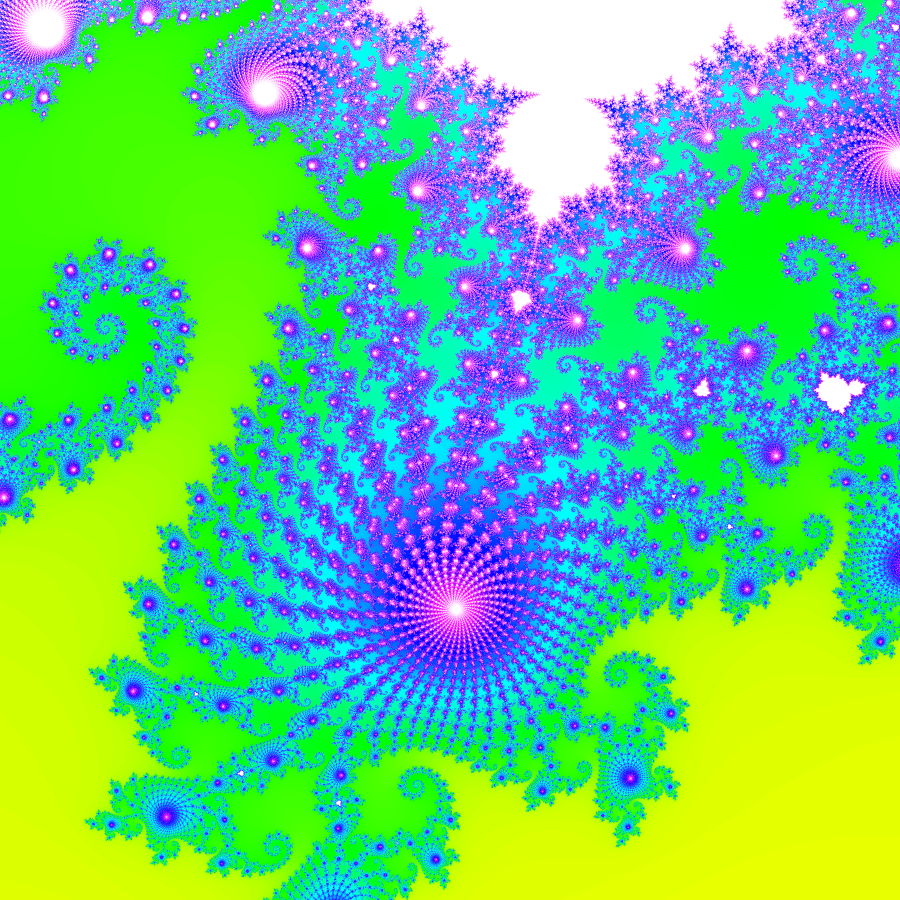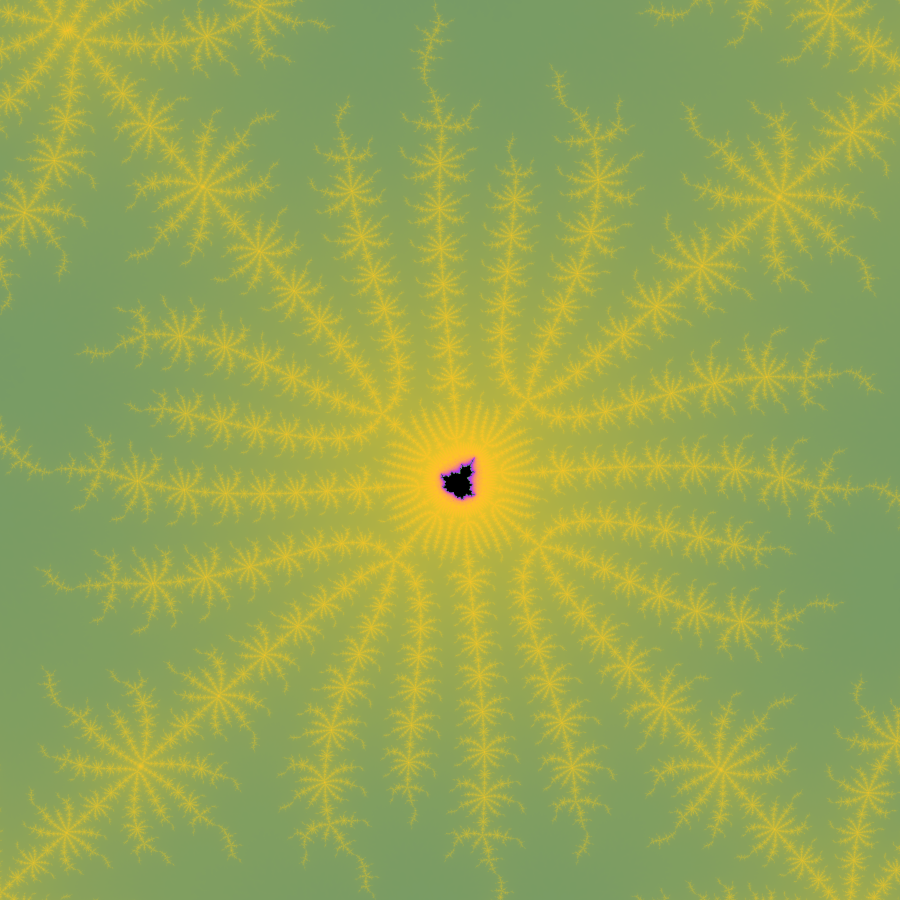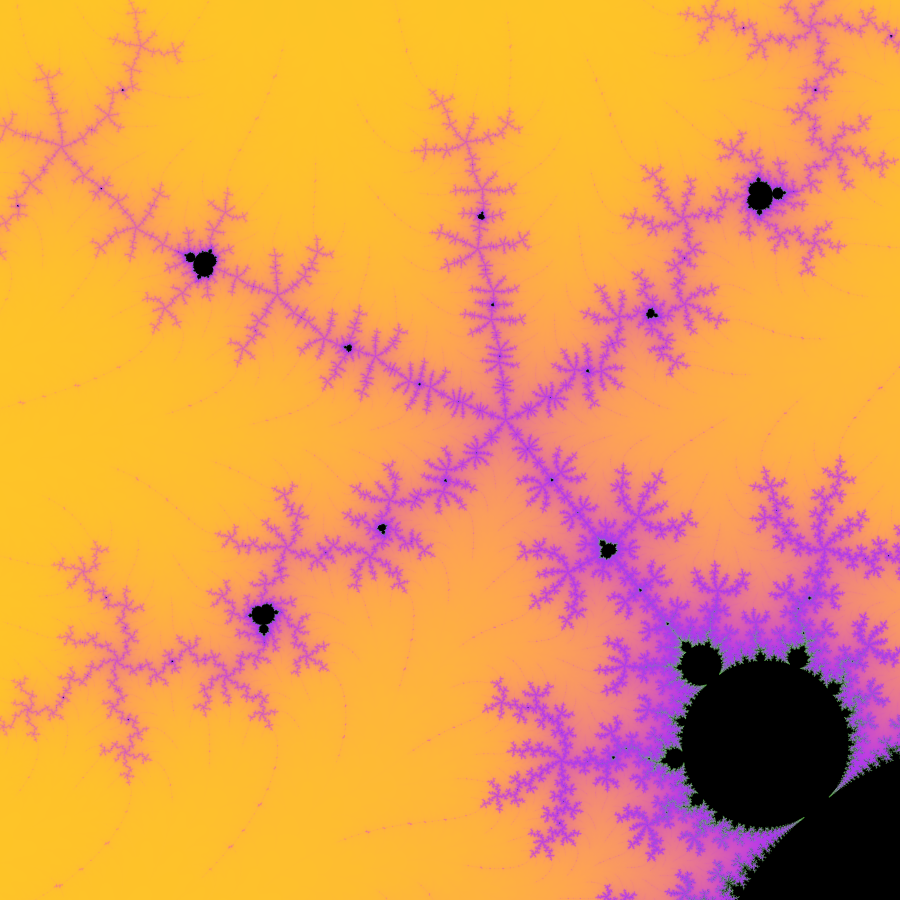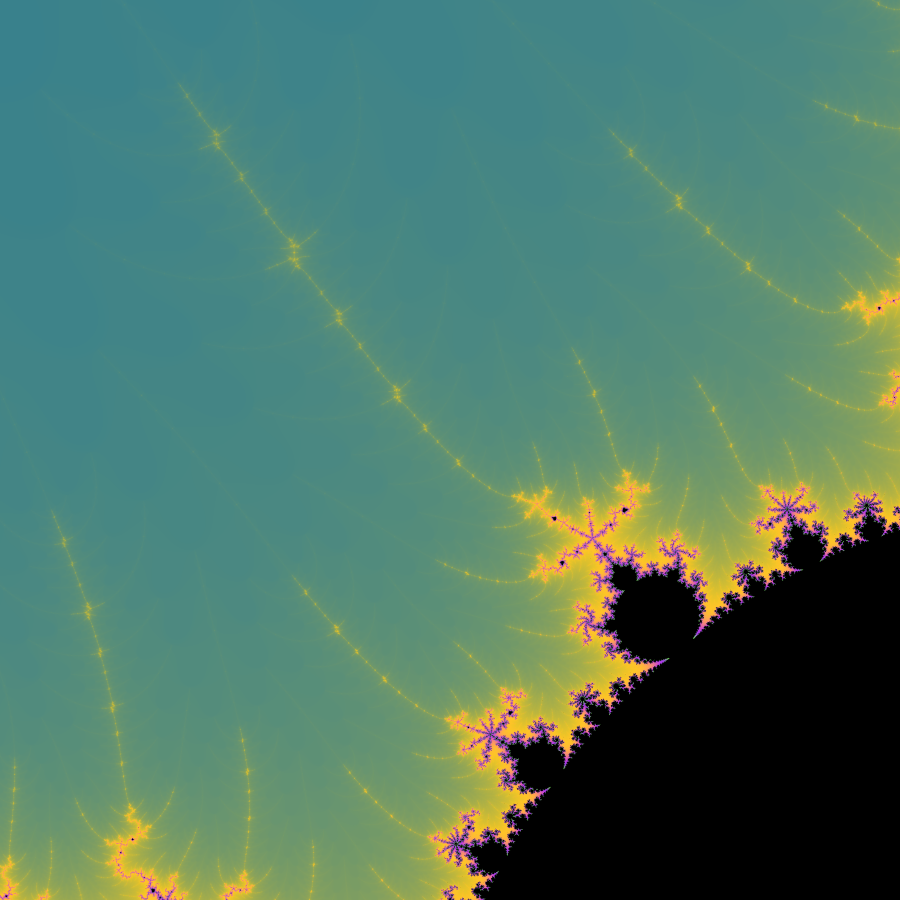## Mandelbrot Variation Fractals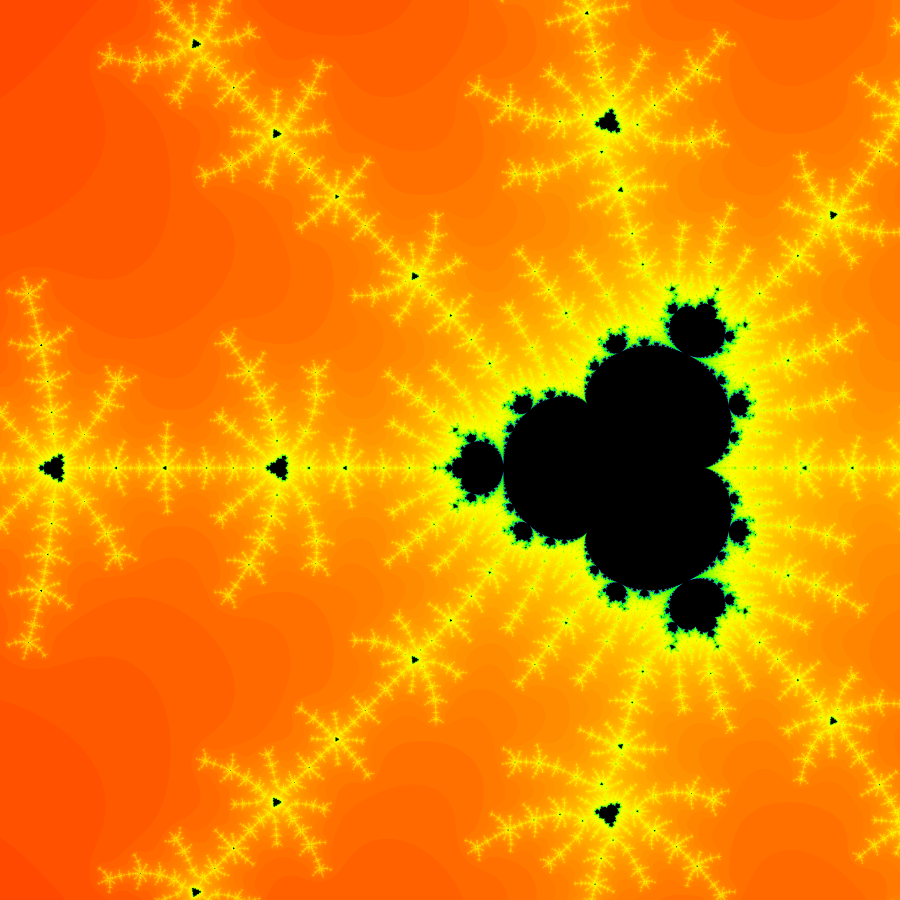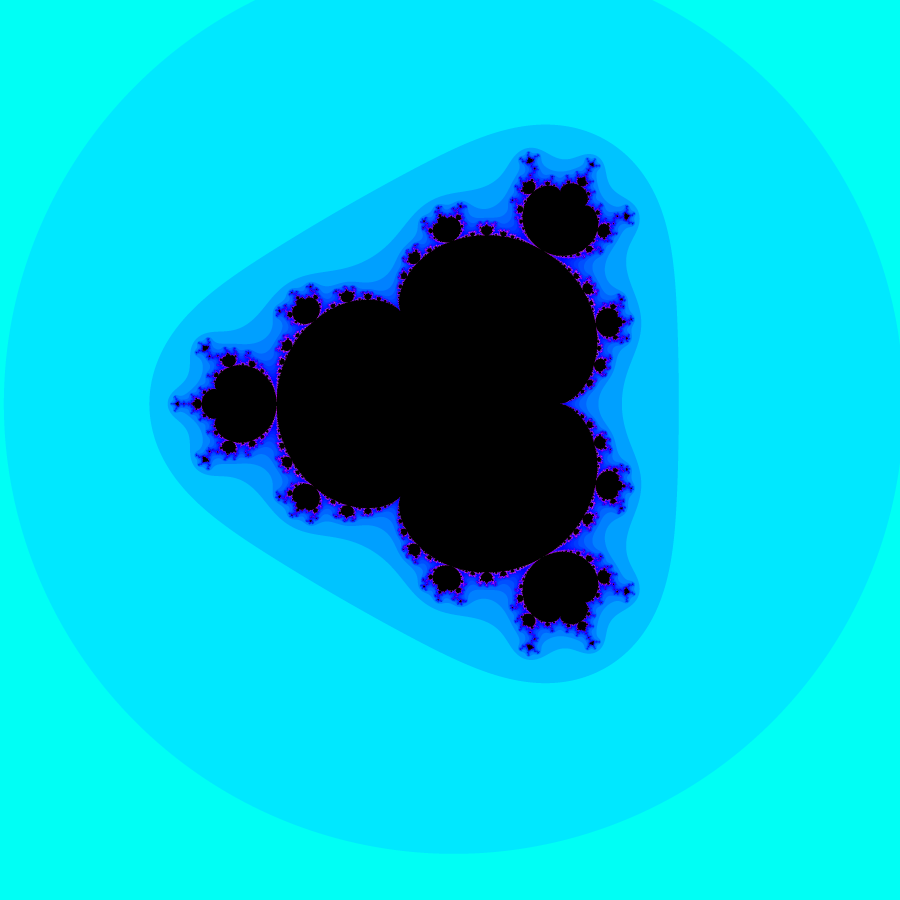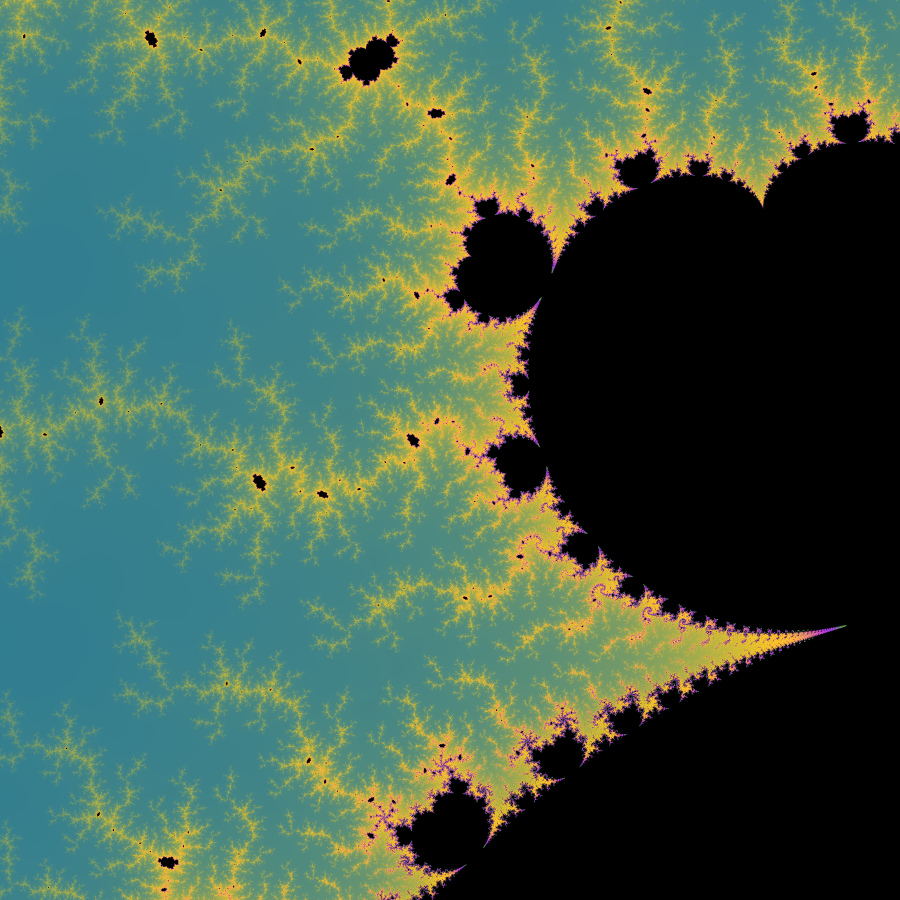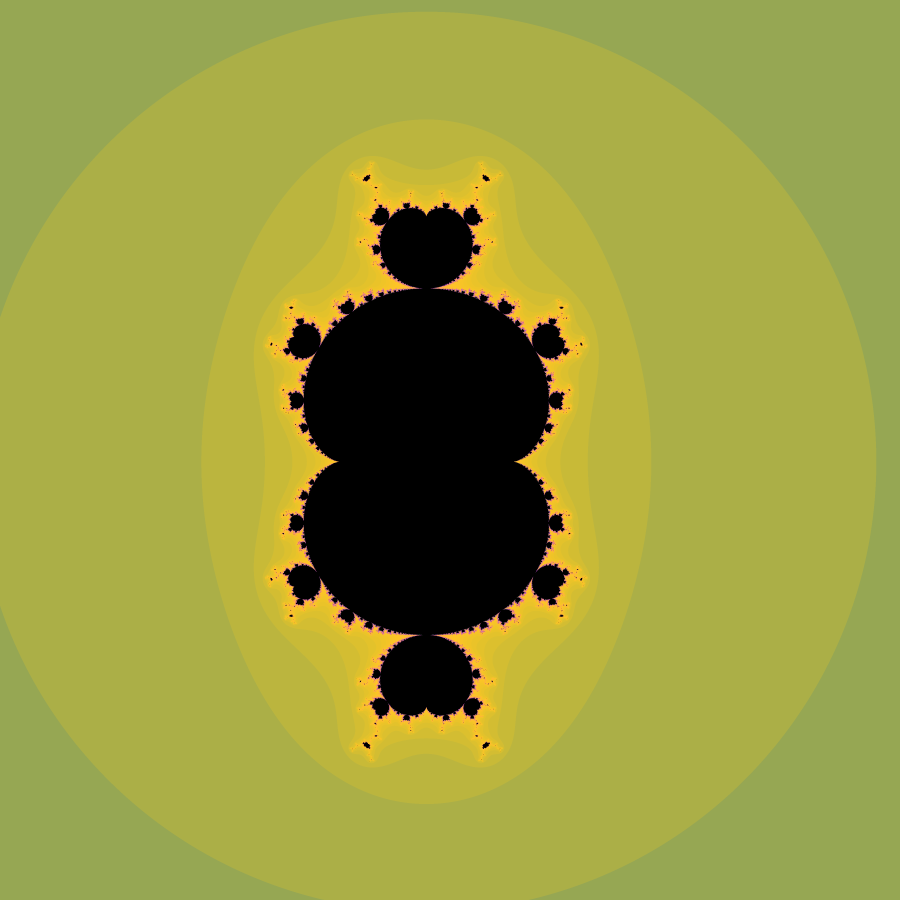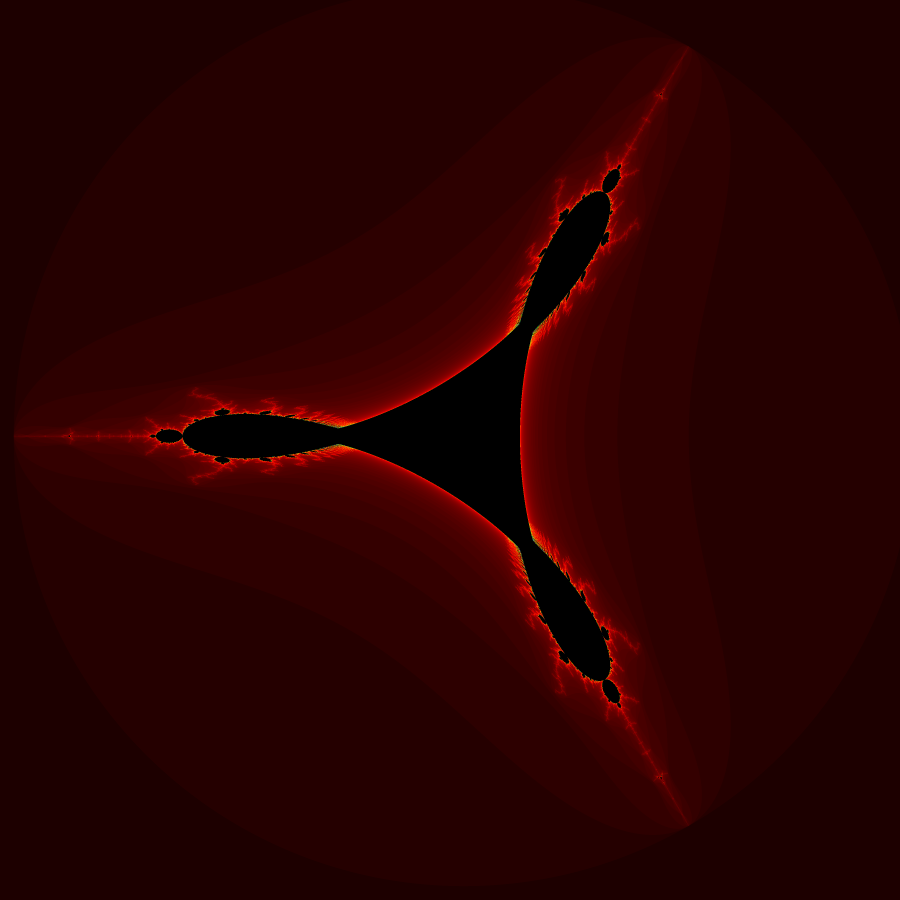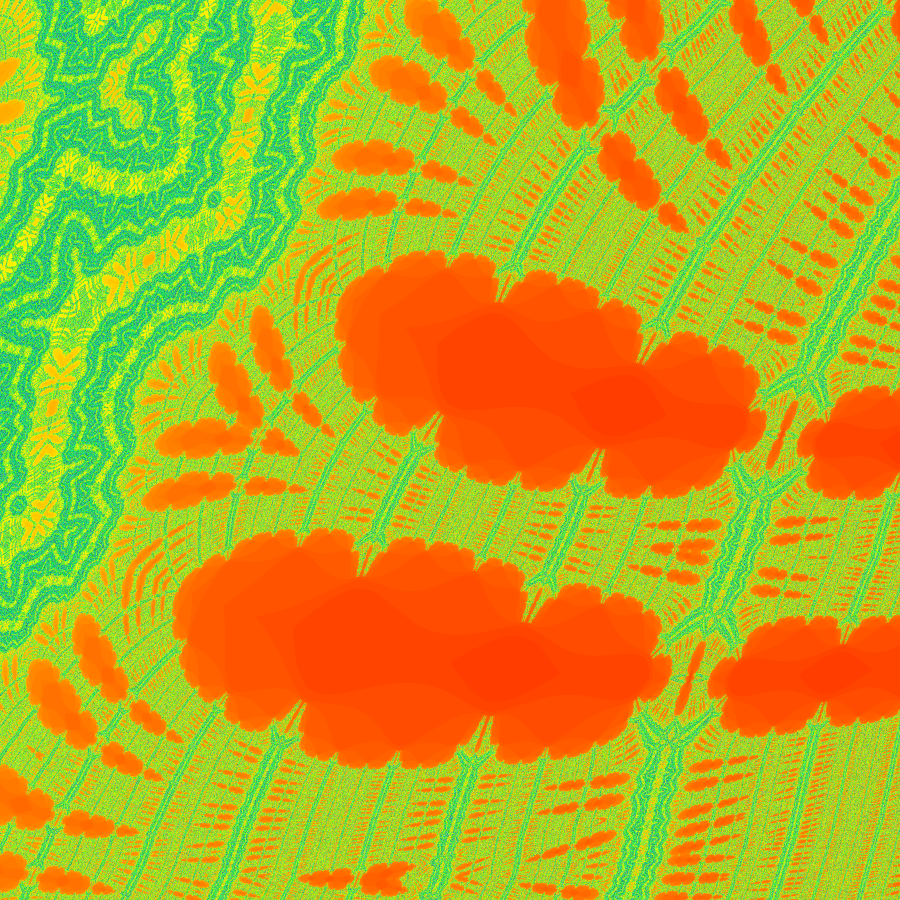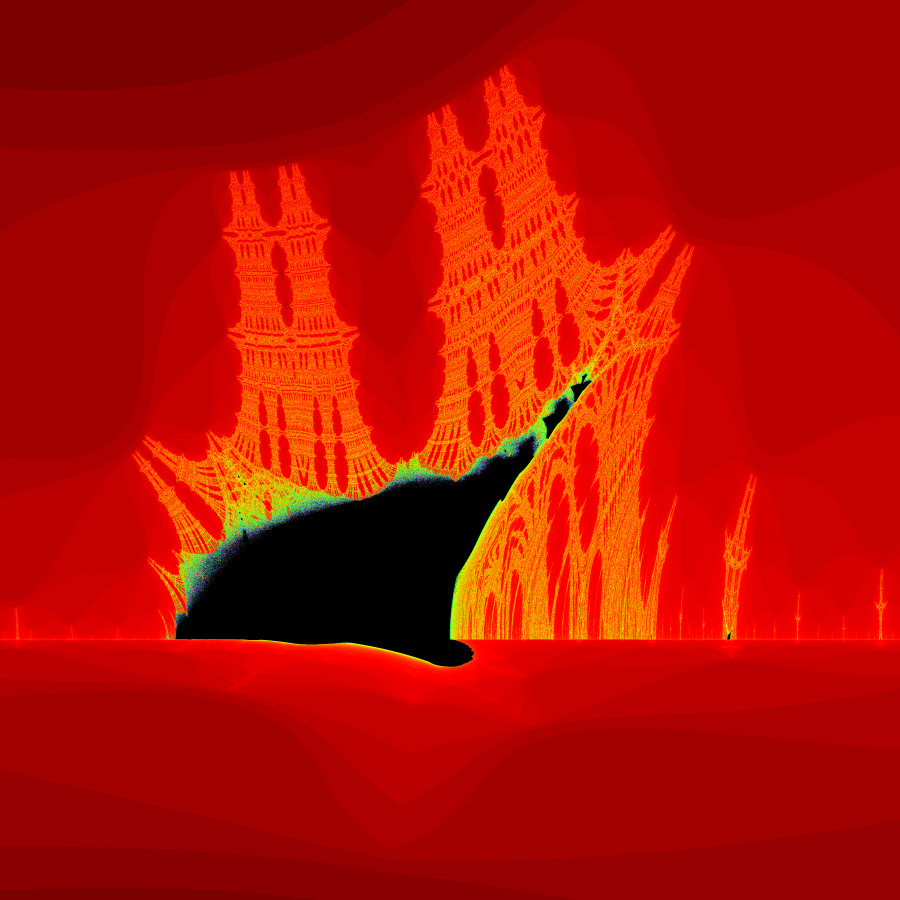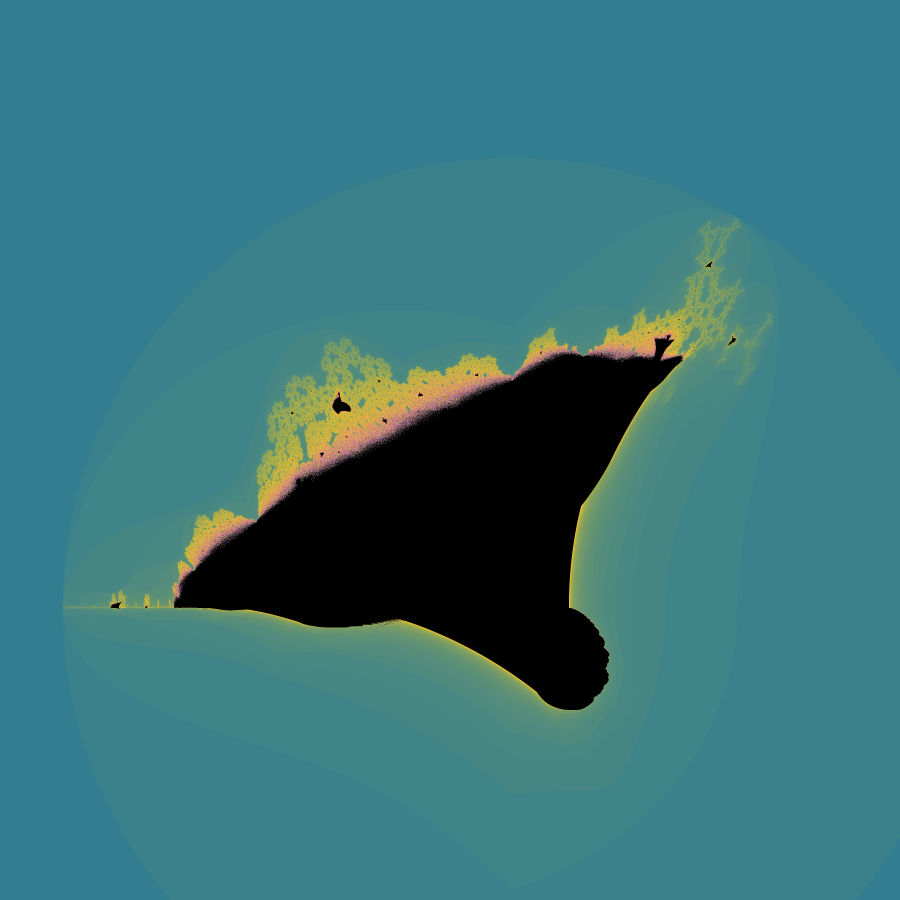## Buddhabrot Fractals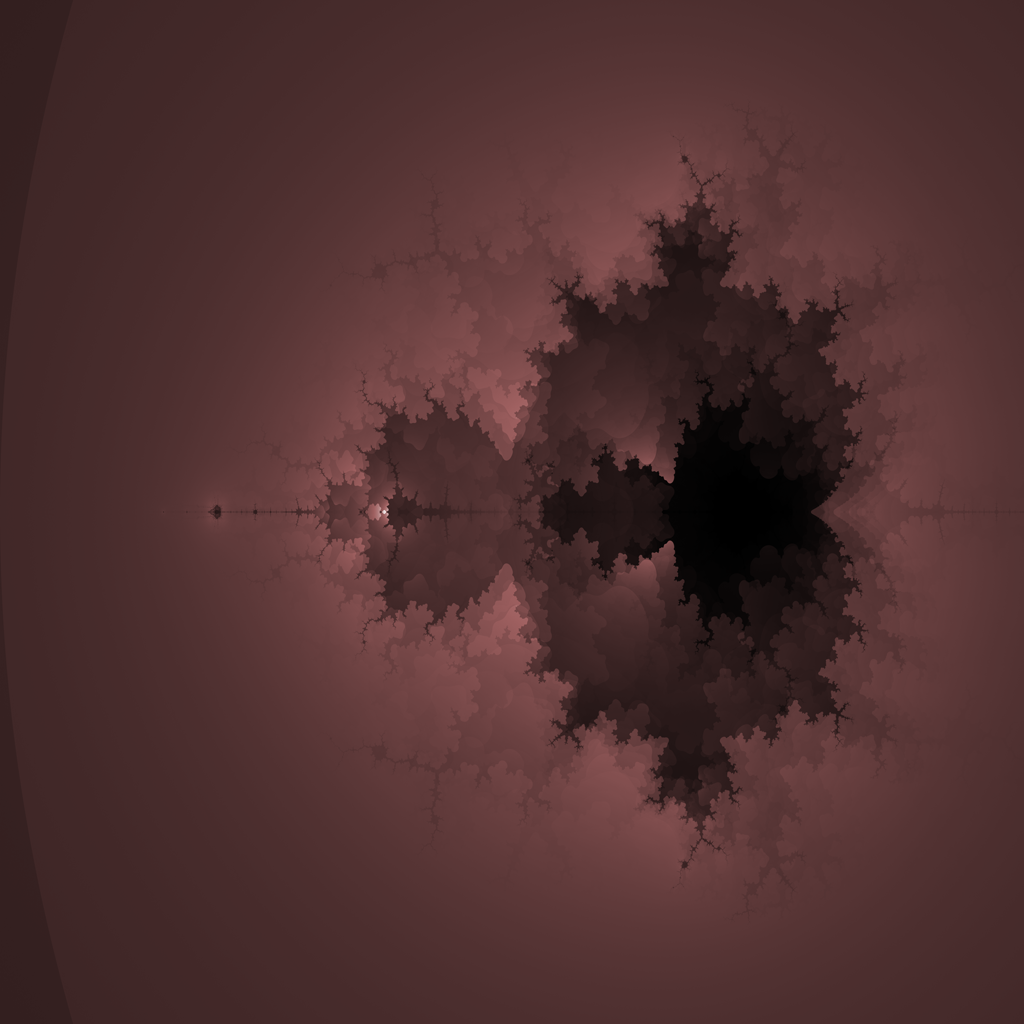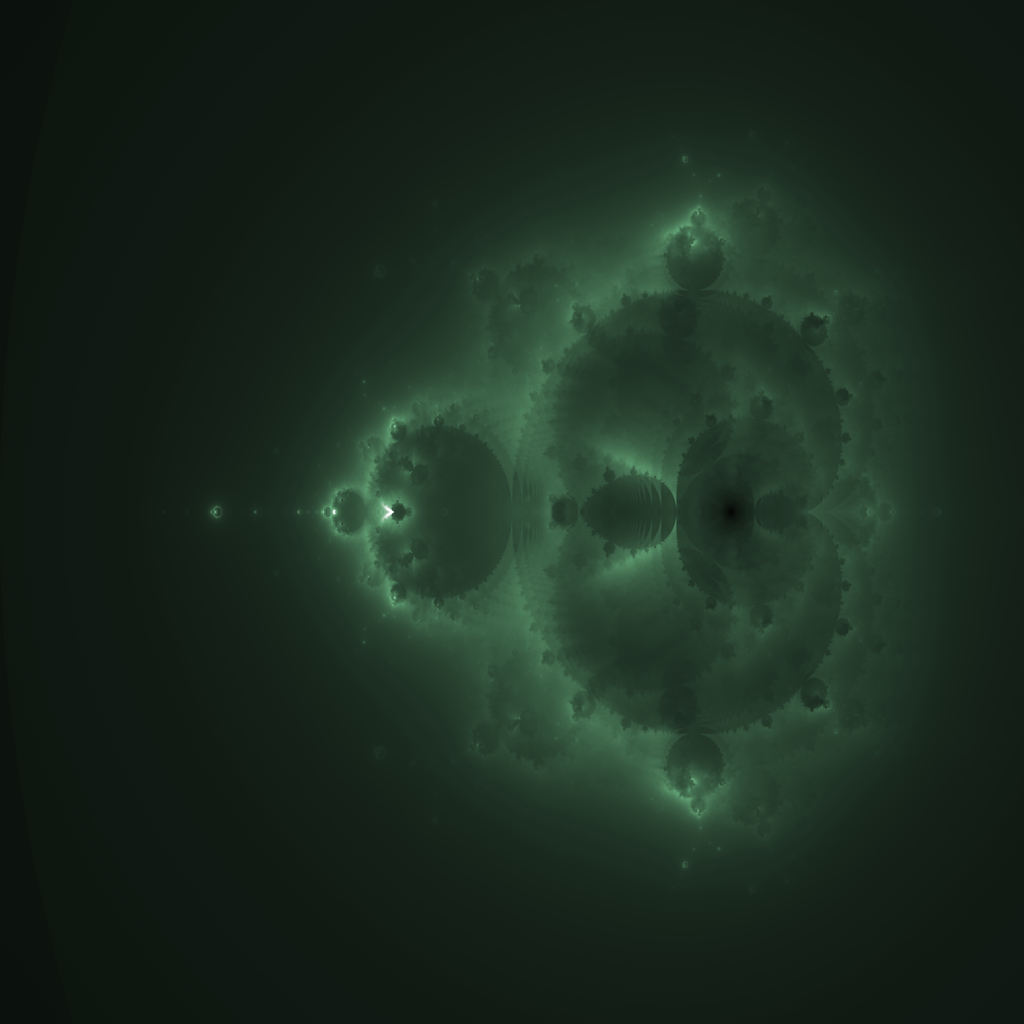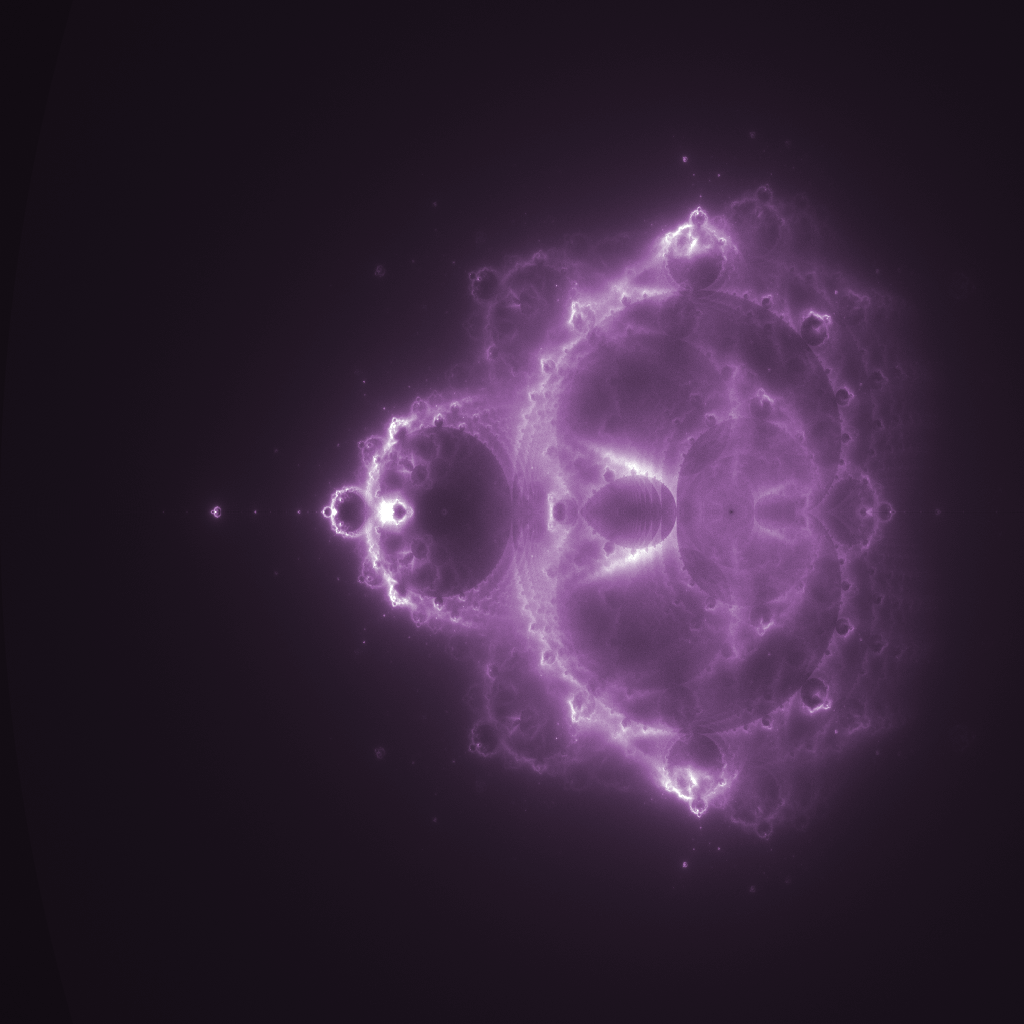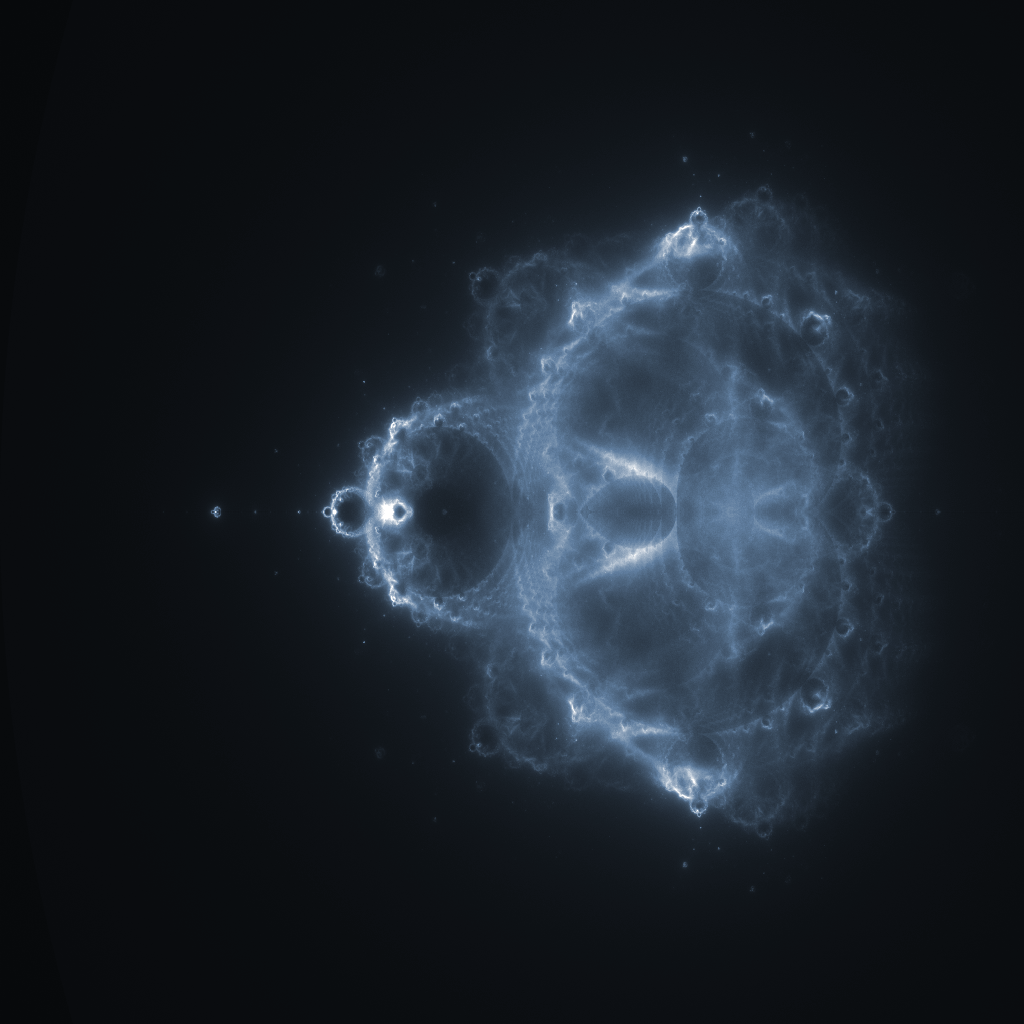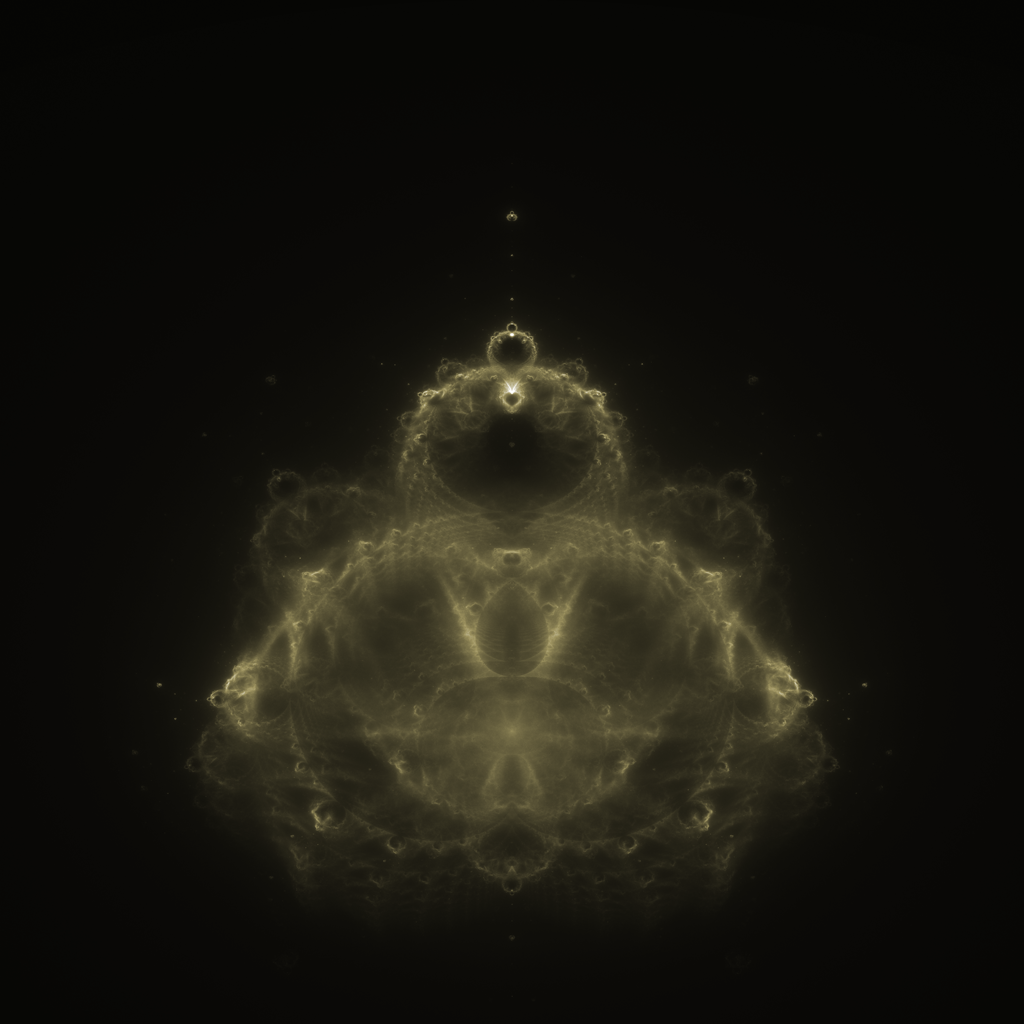## AlloyRender Dual Rasterizing System